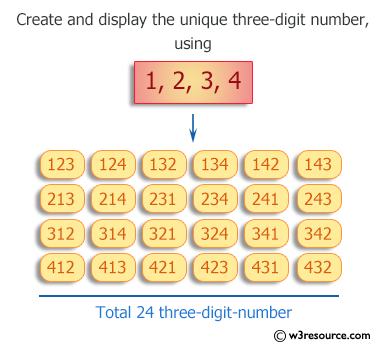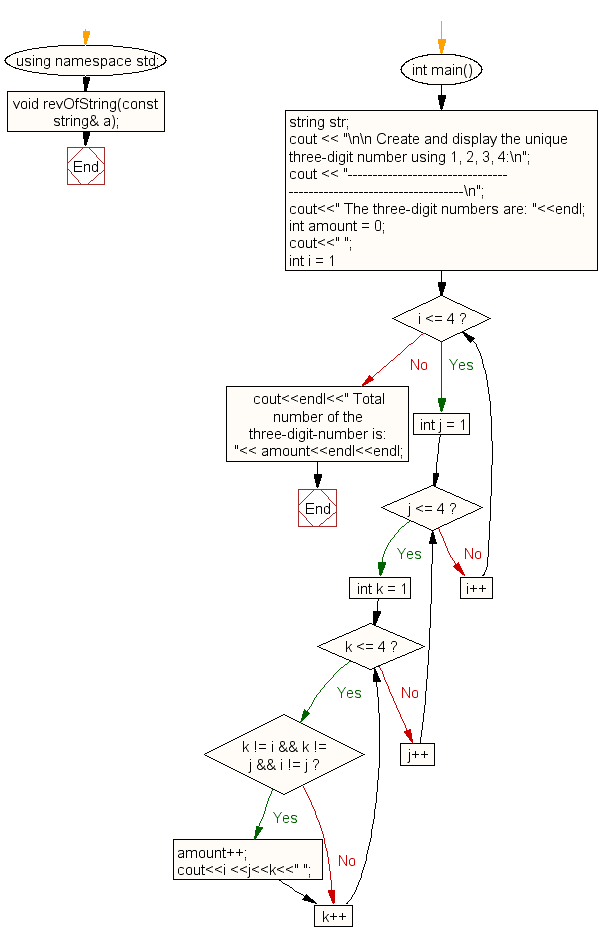﻿ C++ : Create unique three-digit numbers using 1,2,3,4# C++ Exercises: Create and display unique three-digit number using 1, 2, 3, 4

## C++ For Loop: Exercise-87 with Solution

Write a program in C++ to create and display unique three-digit number using 1, 2, 3, 4. Also count how many three-digit numbers are there.

Pictorial Presentation:Sample Solution:-

C++ Code :

``````#include <iostream>
using namespace std;

void revOfString(const string& a);

int main()
{
string str;
cout << "\n\n Create and display the unique three-digit number using 1, 2, 3, 4:\n";
cout << "-------------------------------------------------------------------\n";
cout<<" The three-digit numbers are: "<<endl;
int amount = 0;
cout<<" ";
for(int i = 1; i <= 4; i++)
{
for(int j = 1; j <= 4; j++)
{
for(int k = 1; k <= 4; k++)
{
if(k != i && k != j && i != j)
{
amount++;
cout<<i <<j<<k<<" ";
}
}
}
}
cout<<endl<<" Total number of the three-digit-number is: "<< amount<<endl<<endl;
}
``````

Sample Output:

``` Create and display the unique three-digit number using 1, 2, 3, 4:
-------------------------------------------------------------------
The three-digit numbers are:
123 124 132 134 142 143 213 214 231 234 241 243 312 314 321 324 341 34
2 412 413 421 423 431 432
Total number of the three-digit-number is: 24
```

Flowchart:C++ Code Editor:

Contribute your code and comments through Disqus.

What is the difficulty level of this exercise?

﻿

## C++ Programming: Tips of the Day

What is a smart pointer and when should I use one?

This answer is rather old, and so describes what was 'good' at the time, which was smart pointers provided by the Boost library. Since C++11, the standard library has provided sufficient smart pointers types, and so you should favour the use of std::unique_ptr, std::shared_ptr and std::weak_ptr.

There was also std::auto_ptr. It was very much like a scoped pointer, except that it also had the "special" dangerous ability to be copied - which also unexpectedly transfers ownership.

It was deprecated in C++11 and removed in C++17, so you shouldn't use it.

```std::auto_ptr<MyObject> p1 (new MyObject());
std::auto_ptr<MyObject> p2 = p1; // Copy and transfer ownership.
// p1 gets set to empty!
p2->DoSomething(); // Works.
p1->DoSomething(); // Oh oh. Hopefully raises some NULL pointer exception.
```

Ref : https://bit.ly/3mc9GHE